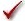## Please enable JavaScript to view this site.

 Navigation: PRINCIPLES OF STATISTICS > Analysis checklists Analysis checklist: Paired t test

The paired t test compares the means of two matched groups, assuming that the distribution of the before-after differences follows a Gaussian distribution.

###Are the differences distributed according to a Gaussian distribution?

The paired t test assumes that you have sampled your pairs of values from a population of pairs where the difference between pairs follows a Gaussian distribution.

While this assumption is not too important with large samples, it is important with small sample sizes. Test this assumption with Prism.

Note that the paired t test, unlike the unpaired t test, does not assume that the two sets of data (before and after, in the typical example) are sampled from populations with equal variances.

###Was the pairing effective?

The pairing should be part of the experimental design and not something you do after collecting data. Prism tests the effectiveness of pairing by calculating the Pearson correlation coefficient, r, and a corresponding P value. If the P value is small, the two groups are significantly correlated. This justifies the use of a paired test.

If this P value is large (say larger than 0.05), you should question whether it made sense to use a paired test. Your choice of whether to use a paired test or not should not be based solely on this one P value, but also on the experimental design and the results of other similar experiments.

###Are the pairs independent?

The results of a paired t test only make sense when the pairs are independent – that whatever factor caused a difference (between paired values) to be too high or too low affects only that one pair. Prism cannot test this assumption. You must think about the experimental design. For example, the errors are not independent if you have six pairs of values, but these were obtained from three animals, with duplicate measurements in each animal. In this case, some factor may cause the after-before differences from one animal to be high or low. This factor would affect two of the pairs, so they are not independent.

###Are you comparing exactly two groups?

Use the t test only to compare two groups. To compare three or more matched groups, use repeated measures one-way ANOVA followed by post tests. It is not appropriate to perform several t tests, comparing two groups at a time.

###If you chose a one-tail P value, did you predict correctly?

If you chose a one-tail P value, you should have predicted which group would have the larger mean before collecting data. Prism does not ask you to record this prediction, but assumes that it is correct. If your prediction was wrong, then ignore the reported P value and state that P>0.50.

###Do you care about differences or ratios?

The paired t test analyzes the differences between pairs. With some experiments, you may observe a very large variability among the differences. The differences are larger when the control value is larger. With these data, you'll get more consistent results if you perform a ratio t test.